# RS Aggarwal Solutions for Class 12 Maths Chapter 1: Relations Exercise 1B

A relation is said to be equivalent if it is reflexive, symmetric and transitive. This is the main concept which is covered under exercise 1B of chapter 1. The solutions for these problems are prepared in a comprehensive manner, which improves problem solving capacity of students. Class 12 is the major turning point for the students to help them shape and achieve their future goals. For more knowledge about the concepts which are discussed in this exercise, students can download RS Aggarwal Solutions Class 12 Maths Chapter 1 Relations Exercise 1B PDF, which are given below.

## RS Aggarwal Solutions for Class 12 Maths Chapter 1: Relations Exercise 1B Download PDF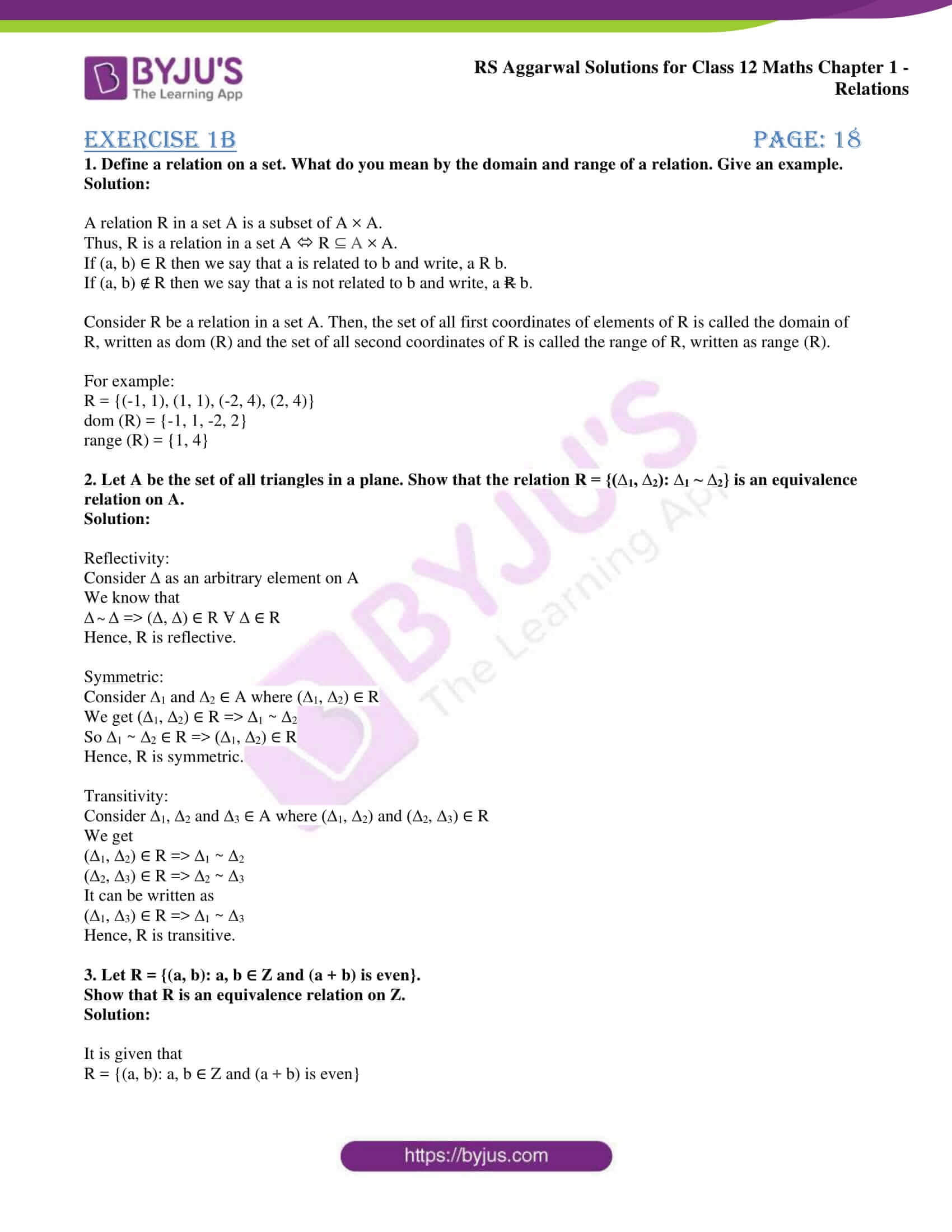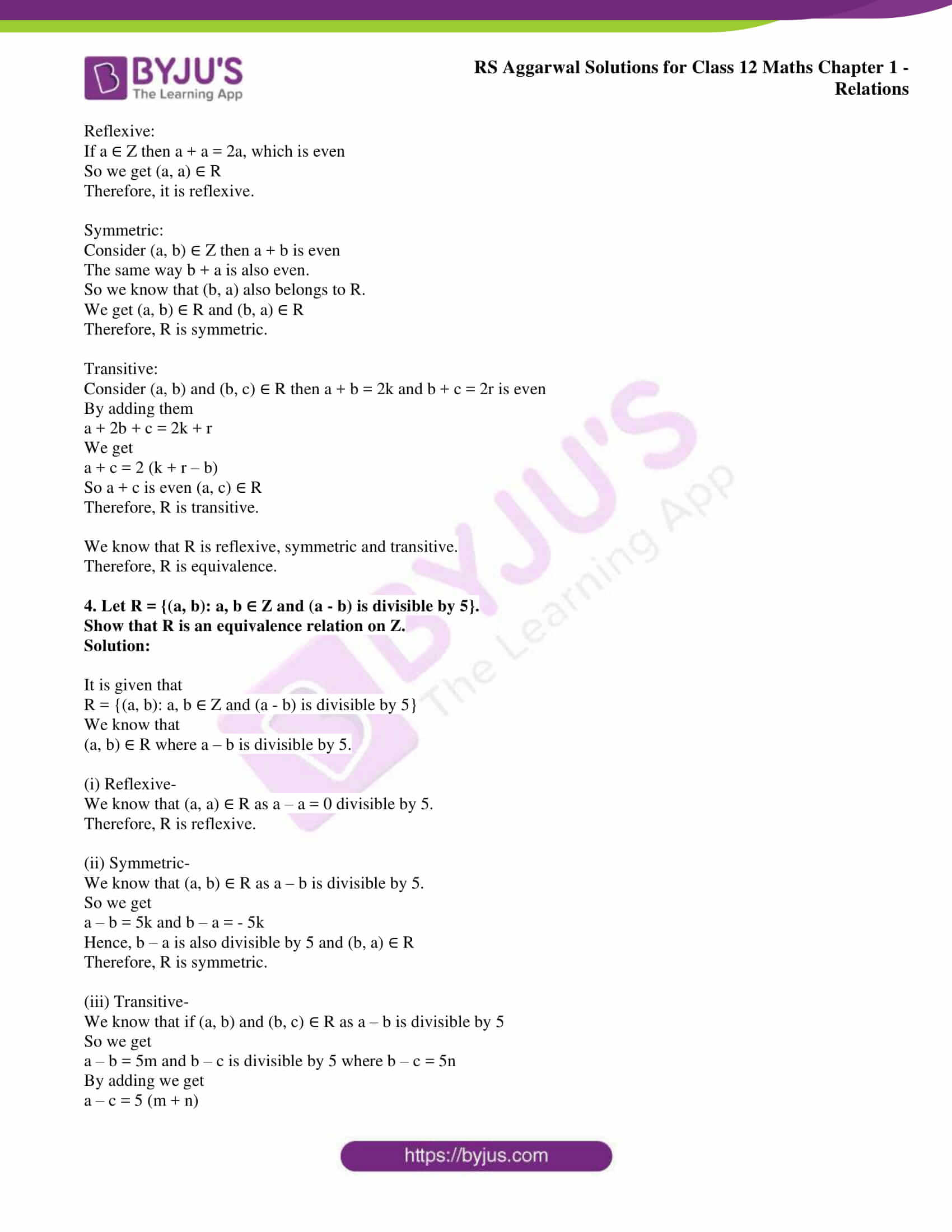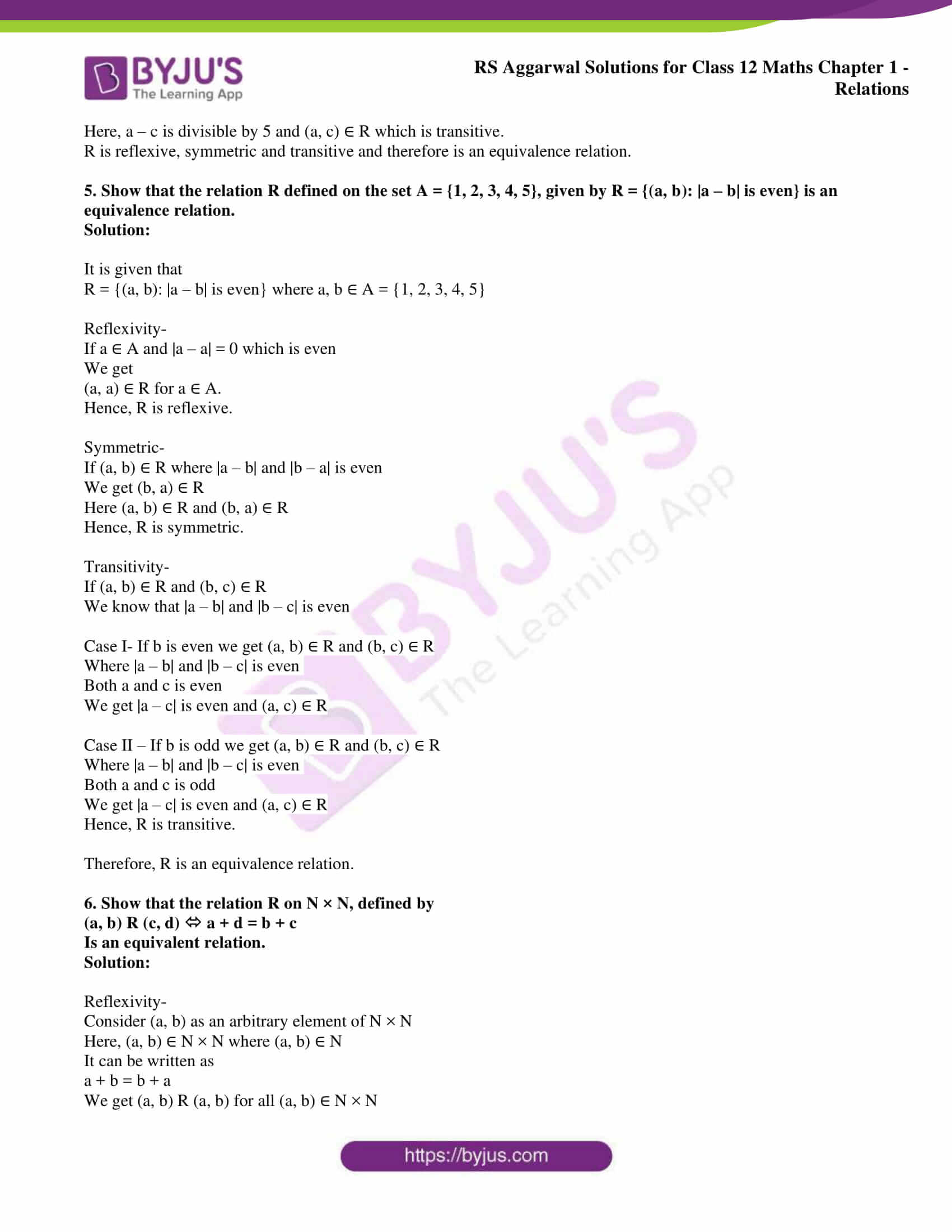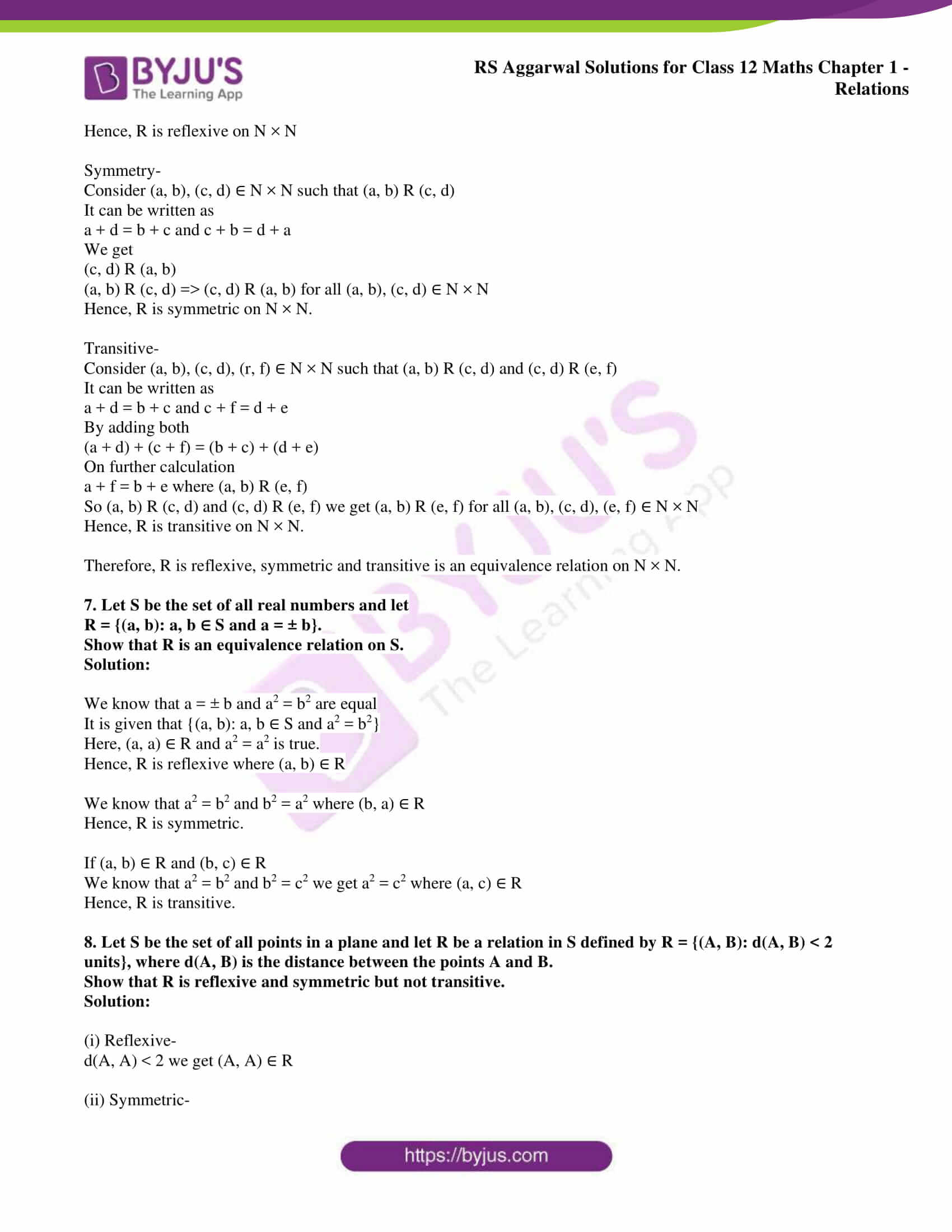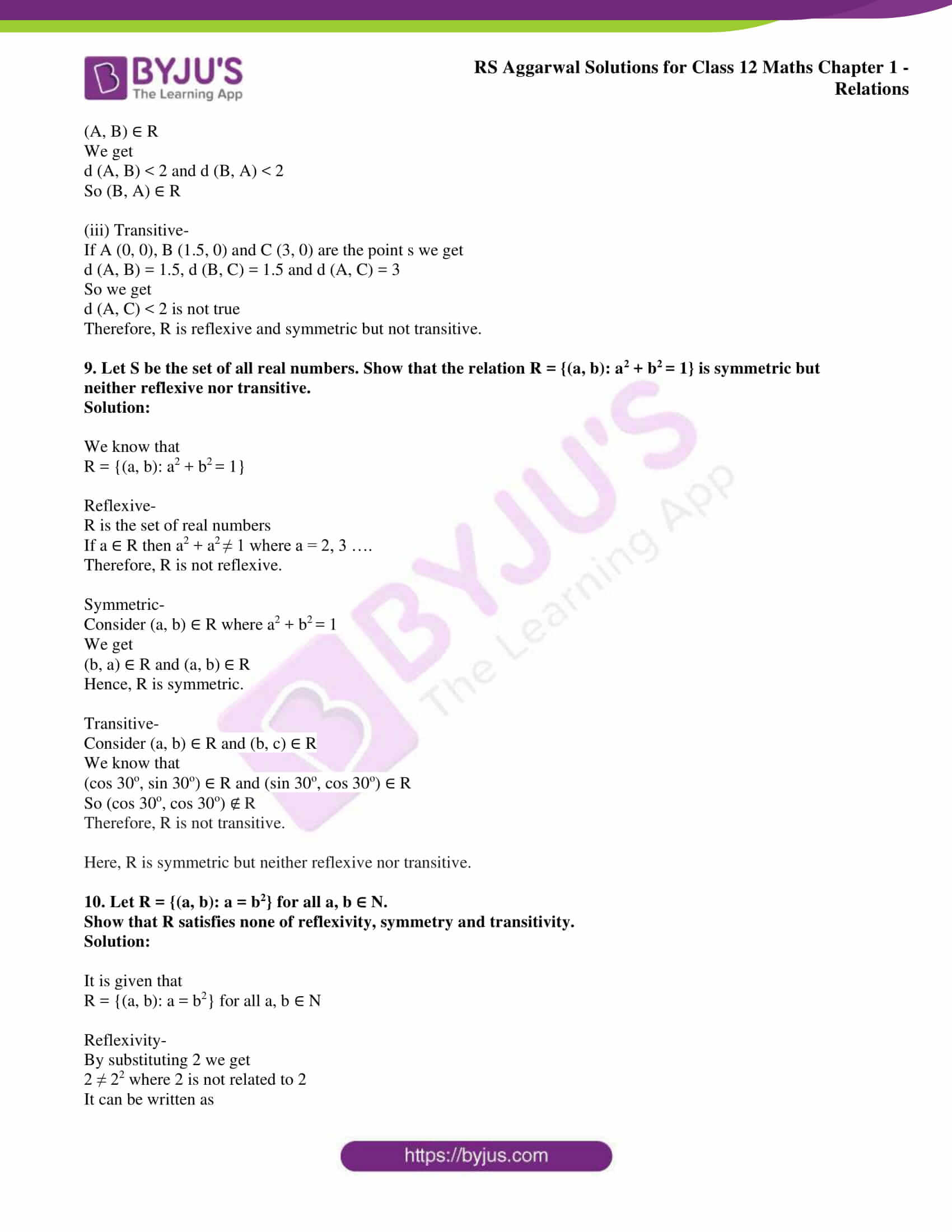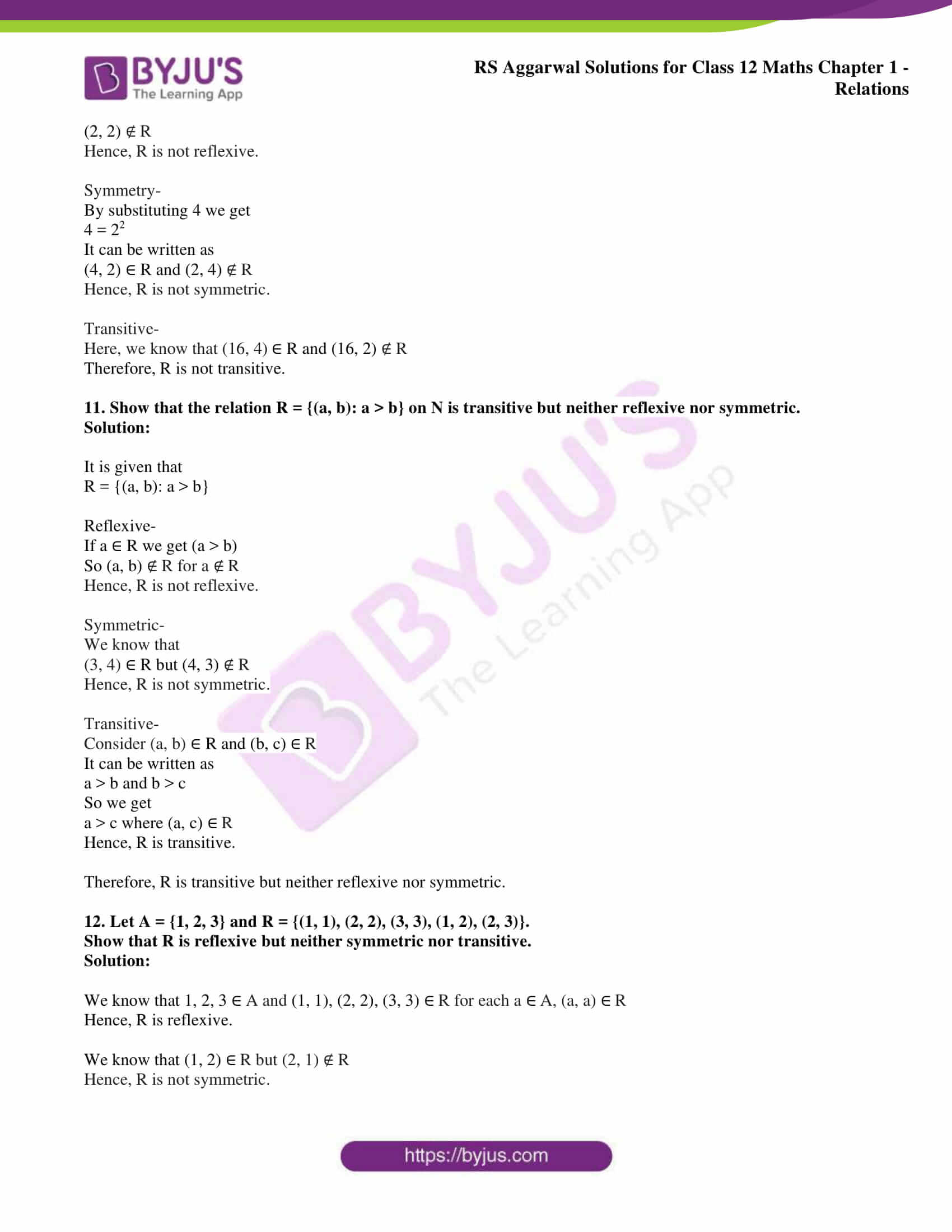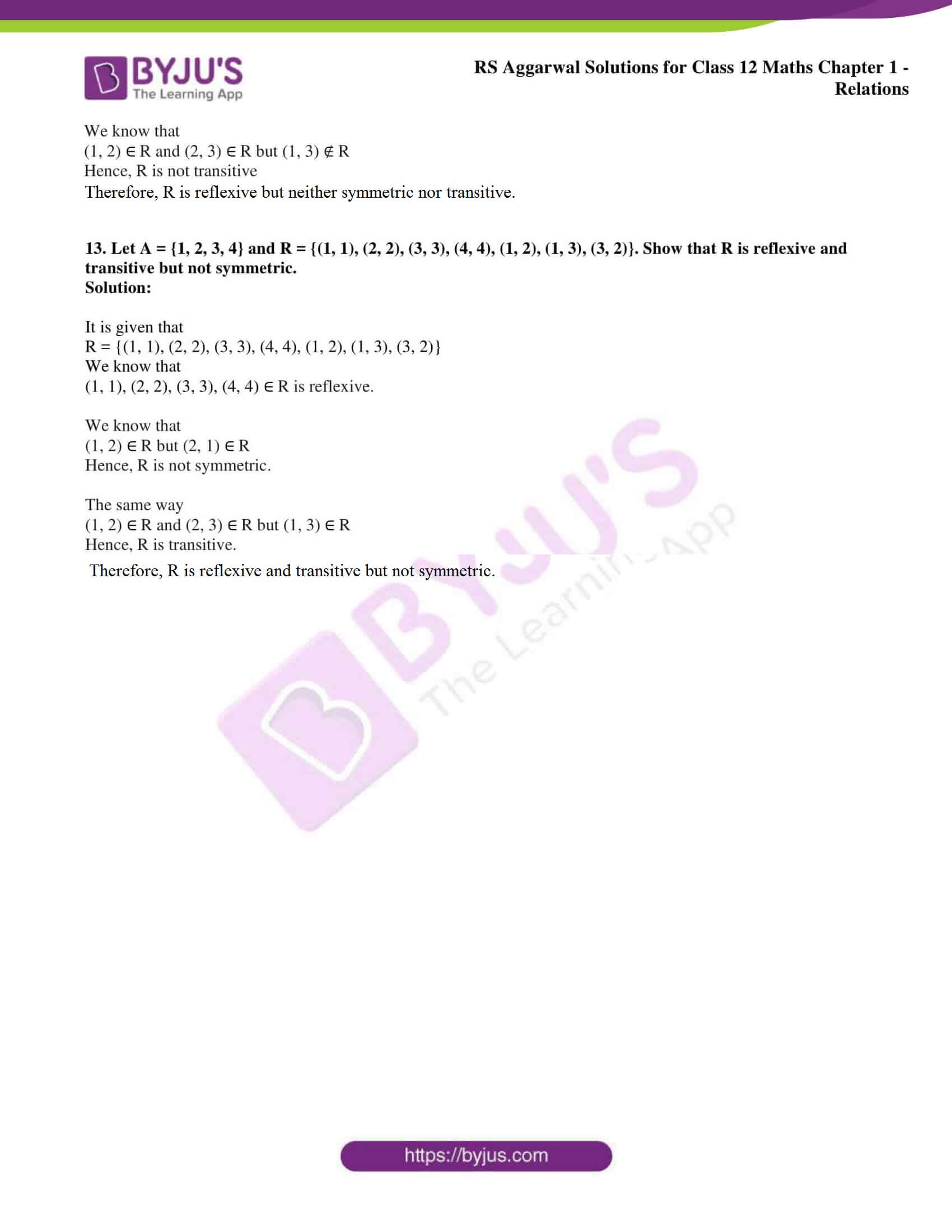### Access other exercise solutions of Class 12 Maths Chapter 1: Relations

Exercise 1A Solutions

### Access RS Aggarwal Solutions for Class 12 Maths Chapter 1: Relations Exercise 1B

1. Define a relation on a set. What do you mean by the domain and range of a relation. Give an example.

Solution:

A relation R in a set A is a subset of A × A.

Thus, R is a relation in a set A R ⊆ A × A.

If (a, b) ∈ R then we say that a is related to b and write, a R b.

If (a, b) ∉ R then we say that a is not related to b and write, a b.

Consider R be a relation in a set A. Then, the set of all first coordinates of elements of R is called the domain of R, written as dom (R) and the set of all second coordinates of R is called the range of R, written as range (R).

For example:

R = {(-1, 1), (1, 1), (-2, 4), (2, 4)}

dom (R) = {-1, 1, -2, 2}

range (R) = {1, 4}

2. Let A be the set of all triangles in a plane. Show that the relation R = {(∆1, ∆2): ∆1 ~ ∆2} is an equivalence relation on A.

Solution:

Reflectivity:

Consider ∆ as an arbitrary element on A

We know that

∆ ~ ∆ => (∆, ∆) ∈ R Ɐ ∆ ∈ R

Hence, R is reflective.

Symmetric:

Consider ∆1 and ∆2 ∈ A where (∆1, ∆2) ∈ R

We get (∆1, ∆2) ∈ R => ∆1 ~ ∆2

So ∆1 ~ ∆2 ∈ R => (∆1, ∆2) ∈ R

Hence, R is symmetric.

Transitivity:

Consider ∆1, ∆2 and ∆3 ∈ A where (∆1, ∆2) and (∆2, ∆3) ∈ R

We get

(∆1, ∆2) ∈ R => ∆1 ~ ∆2

(∆2, ∆3) ∈ R => ∆2 ~ ∆3

It can be written as

(∆1, ∆3) ∈ R => ∆1 ~ ∆3

Hence, R is transitive.

3. Let R = {(a, b): a, b ∈ Z and (a + b) is even}.

Show that R is an equivalence relation on Z.

Solution:

It is given that

R = {(a, b): a, b ∈ Z and (a + b) is even}

Reflexive:

If a ∈ Z then a + a = 2a, which is even

So we get (a, a) ∈ R

Therefore, it is reflexive.

Symmetric:

Consider (a, b) ∈ Z then a + b is even

The same way b + a is also even.

So we know that (b, a) also belongs to R.

We get (a, b) ∈ R and (b, a) ∈ R

Therefore, R is symmetric.

Transitive:

Consider (a, b) and (b, c) ∈ R then a + b = 2k and b + c = 2r is even

a + 2b + c = 2k + r

We get

a + c = 2 (k + r – b)

So a + c is even (a, c) ∈ R

Therefore, R is transitive.

We know that R is reflexive, symmetric and transitive.

Therefore, R is equivalence.

4. Let R = {(a, b): a, b ∈ Z and (a – b) is divisible by 5}.

Show that R is an equivalence relation on Z.

Solution:

It is given that

R = {(a, b): a, b ∈ Z and (a – b) is divisible by 5}

We know that

(a, b) ∈ R where a – b is divisible by 5.

(i) Reflexive-

We know that (a, a) ∈ R as a – a = 0 divisible by 5.

Therefore, R is reflexive.

(ii) Symmetric-

We know that (a, b) ∈ R as a – b is divisible by 5.

So we get

a – b = 5k and b – a = – 5k

Hence, b – a is also divisible by 5 and (b, a) ∈ R

Therefore, R is symmetric.

(iii) Transitive-

We know that if (a, b) and (b, c) ∈ R as a – b is divisible by 5

So we get

a – b = 5m and b – c is divisible by 5 where b – c = 5n

a – c = 5 (m + n)

Here, a – c is divisible by 5 and (a, c) ∈ R which is transitive.

R is reflexive, symmetric and transitive and therefore is an equivalence relation.

5. Show that the relation R defined on the set A = {1, 2, 3, 4, 5}, given by R = {(a, b): |a – b| is even} is an equivalence relation.

Solution:

It is given that

R = {(a, b): |a – b| is even} where a, b ∈ A = {1, 2, 3, 4, 5}

Reflexivity-

If a ∈ A and |a – a| = 0 which is even

We get

(a, a) ∈ R for a ∈ A.

Hence, R is reflexive.

Symmetric-

If (a, b) ∈ R where |a – b| and |b – a| is even

We get (b, a) ∈ R

Here (a, b) ∈ R and (b, a) ∈ R

Hence, R is symmetric.

Transitivity-

If (a, b) ∈ R and (b, c) ∈ R

We know that |a – b| and |b – c| is even

Case I- If b is even we get (a, b) ∈ R and (b, c) ∈ R

Where |a – b| and |b – c| is even

Both a and c is even

We get |a – c| is even and (a, c) ∈ R

Case II – If b is odd we get (a, b) ∈ R and (b, c) ∈ R

Where |a – b| and |b – c| is even

Both a and c is odd

We get |a – c| is even and (a, c) ∈ R

Hence, R is transitive.

Therefore, R is an equivalence relation.

6. Show that the relation R on N × N, defined by

(a, b) R (c, d) a + d = b + c

Is an equivalent relation.

Solution:

Reflexivity-

Consider (a, b) as an arbitrary element of N × N

Here, (a, b) ∈ N × N where (a, b) ∈ N

It can be written as

a + b = b + a

We get (a, b) R (a, b) for all (a, b) ∈ N × N

Hence, R is reflexive on N × N

Symmetry-

Consider (a, b), (c, d) ∈ N × N such that (a, b) R (c, d)

It can be written as

a + d = b + c and c + b = d + a

We get

(c, d) R (a, b)

(a, b) R (c, d) => (c, d) R (a, b) for all (a, b), (c, d) ∈ N × N

Hence, R is symmetric on N × N.

Transitive-

Consider (a, b), (c, d), (r, f) ∈ N × N such that (a, b) R (c, d) and (c, d) R (e, f)

It can be written as

a + d = b + c and c + f = d + e

(a + d) + (c + f) = (b + c) + (d + e)

On further calculation

a + f = b + e where (a, b) R (e, f)

So (a, b) R (c, d) and (c, d) R (e, f) we get (a, b) R (e, f) for all (a, b), (c, d), (e, f) ∈ N × N

Hence, R is transitive on N × N.

Therefore, R is reflexive, symmetric and transitive is an equivalence relation on N × N.

7. Let S be the set of all real numbers and let

R = {(a, b): a, b ∈ S and a = ± b}.

Show that R is an equivalence relation on S.

Solution:

We know that a = ± b and a2 = b2 are equal

It is given that {(a, b): a, b ∈ S and a2 = b2}

Here, (a, a) ∈ R and a2 = a2 is true.

Hence, R is reflexive where (a, b) ∈ R

We know that a2 = b2 and b2 = a2 where (b, a) ∈ R

Hence, R is symmetric.

If (a, b) ∈ R and (b, c) ∈ R

We know that a2 = b2 and b2 = c2 we get a2 = c2 where (a, c) ∈ R

Hence, R is transitive.

8. Let S be the set of all points in a plane and let R be a relation in S defined by R = {(A, B): d(A, B) < 2 units}, where d(A, B) is the distance between the points A and B.

Show that R is reflexive and symmetric but not transitive.

Solution:

(i) Reflexive-

d(A, A) < 2 we get (A, A) ∈ R

(ii) Symmetric-

(A, B) ∈ R

We get

d (A, B) < 2 and d (B, A) < 2

So (B, A) ∈ R

(iii) Transitive-

If A (0, 0), B (1.5, 0) and C (3, 0) are the point s we get

d (A, B) = 1.5, d (B, C) = 1.5 and d (A, C) = 3

So we get

d (A, C) < 2 is not true

Therefore, R is reflexive and symmetric but not transitive.

9. Let S be the set of all real numbers. Show that the relation R = {(a, b): a2 + b2 = 1} is symmetric but neither reflexive nor transitive.

Solution:

We know that

R = {(a, b): a2 + b2 = 1}

Reflexive-

R is the set of real numbers

If a ∈ R then a2 + a2 ≠ 1 where a = 2, 3 ….

Therefore, R is not reflexive.

Symmetric-

Consider (a, b) ∈ R where a2 + b2 = 1

We get

(b, a) ∈ R and (a, b) ∈ R

Hence, R is symmetric.

Transitive-

Consider (a, b) ∈ R and (b, c) ∈ R

We know that

(cos 30o, sin 30o) ∈ R and (sin 30o, cos 30o) ∈ R

So (cos 30o, cos 30o) ∉ R

Therefore, R is not transitive.

Here, R is symmetric but neither reflexive nor transitive.

10. Let R = {(a, b): a = b2} for all a, b ∈ N.

Show that R satisfies none of reflexivity, symmetry and transitivity.

Solution:

It is given that

R = {(a, b): a = b2} for all a, b ∈ N

Reflexivity-

By substituting 2 we get

2 ≠ 22 where 2 is not related to 2

It can be written as

(2, 2) ∉ R

Hence, R is not reflexive.

Symmetry-

By substituting 4 we get

4 = 22

It can be written as

(4, 2) ∈ R and (2, 4) ∉ R

Hence, R is not symmetric.

Transitive-

Here, we know that (16, 4) ∈ R and (16, 2) ∉ R

Therefore, R is not transitive.

11. Show that the relation R = {(a, b): a > b} on N is transitive but neither reflexive nor symmetric.

Solution:

It is given that

R = {(a, b): a > b}

Reflexive-

If a ∈ R we get (a > b)

So (a, b) ∉ R for a ∉ R

Hence, R is not reflexive.

Symmetric-

We know that

(3, 4) ∈ R but (4, 3) ∉ R

Hence, R is not symmetric.

Transitive-

Consider (a, b) ∈ R and (b, c) ∈ R

It can be written as

a > b and b > c

So we get

a > c where (a, c) ∈ R

Hence, R is transitive.

Therefore, R is transitive but neither reflexive nor symmetric.

12. Let A = {1, 2, 3} and R = {(1, 1), (2, 2), (3, 3), (1, 2), (2, 3)}.

Show that R is reflexive but neither symmetric nor transitive.

Solution:

We know that 1, 2, 3 ∈ A and (1, 1), (2, 2), (3, 3) ∈ R for each a ∈ A, (a, a) ∈ R

Hence, R is reflexive.

We know that (1, 2) ∈ R but (2, 1) ∉ R

Hence, R is not symmetric.

We know that

(1, 2) ∈ R and (2, 3) ∈ R but (1, 3) ∉ R

Hence, R is not transitive

Therefore, R is reflexive but neither symmetric nor transitive.

13. Let A = {1, 2, 3, 4} and R = {(1, 1), (2, 2), (3, 3), (4, 4), (1, 2), (1, 3), (3, 2)}. Show that R is reflexive and transitive but not symmetric.

Solution:

It is given that

R = {(1, 1), (2, 2), (3, 3), (4, 4), (1, 2), (1, 3), (3, 2)}

We know that

(1, 1), (2, 2), (3, 3), (4, 4) ∈ R is reflexive.

We know that

(1, 2) ∈ R but (2, 1) ∈ R

Hence, R is not symmetric.

The same way

(1, 2) ∈ R and (2, 3) ∈ R but (1, 3) ∈ R

Hence, R is transitive.

Therefore, R is reflexive and transitive but not symmetric.